# BNN Processor (Bayesian Neural Network)

Modified on Tue, 30 Nov 2021 at 03:41 PM

The BNN processor can be used to forecast a ratio-scaled variable based on a neural network which is built using the differential evolution (DE) algorithm in several iterations.

The BNN processor builds several neural networks in several iterations (depending on the configuration settings) and evaluates whether the fitness of all neural networks after each iteration has improved in comparison to the networks of the previous iteration.

The neural networks consist of three layers. An input layer with the input nodes (represented by the independent variables), a hidden layer with a certain number of nodes that can be specified in the configuration, and an output layer which gives the forecast produced by the neural network. The nodes of the neural network are connected by links with certain weights. In each iteration the weights of the neural networks get modified slightly based on the differential evolution algorithm and a random component.

The fitness in each iteration is calculated based on the prediction error across all rows and the complexity of the neural network. A more complex network leads to a worse fitness. This means the processor tries to build a very simple network with a low prediction error.

## Input

The processor requires an input dataset that contains at least one ratio-scaled independent variable and one ratio-scaled dependent variable. The left input node should be connected with the training data, the right input node with the test data.

## Configuration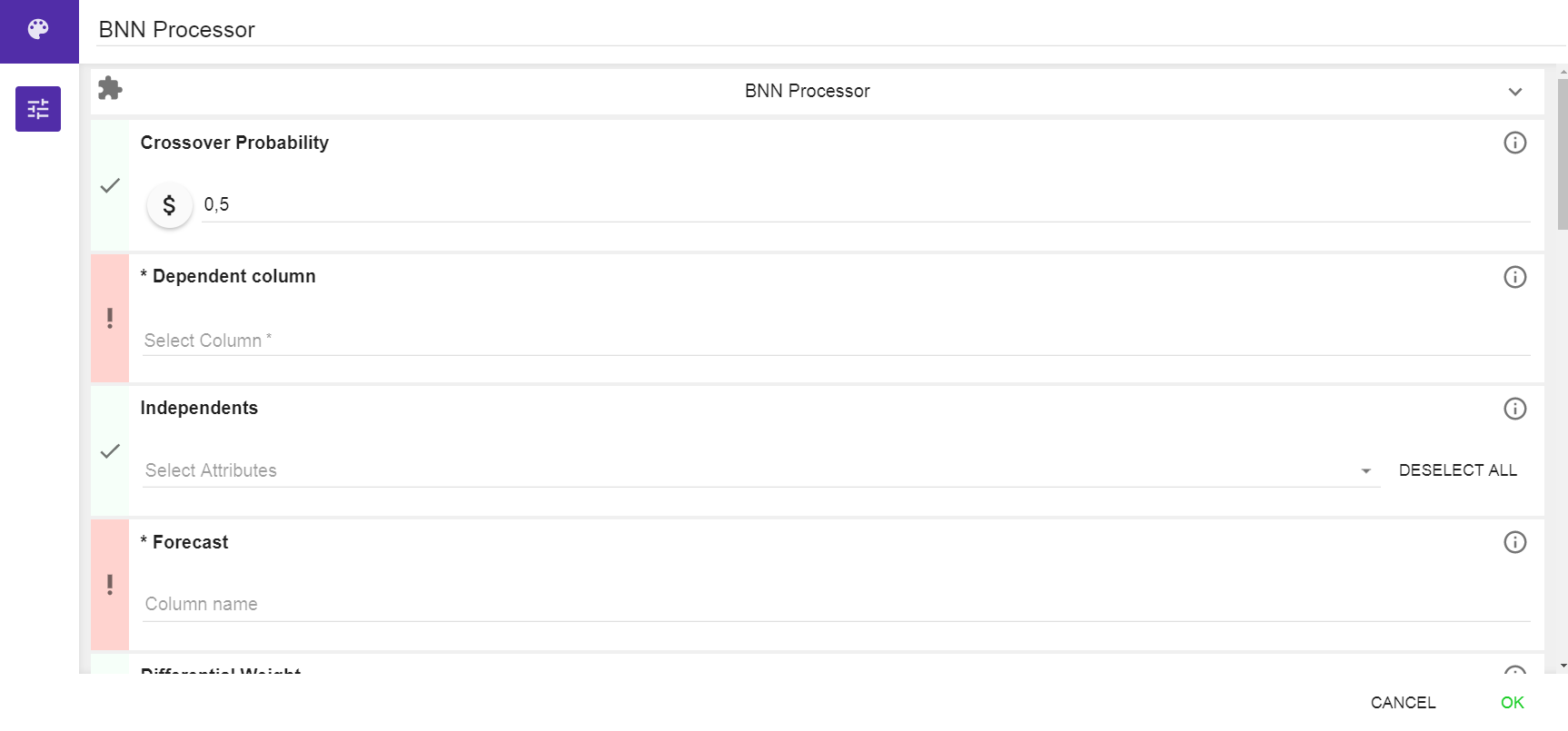Configuration interface

Crossover Probability: Enter a cross-over probability for the differential evolution algorithm. In order to find better networks, the processor combines models that already had a good fitness in order to find models that have an even better fitness. The cross-over probability determines with which probability a cross-over will take place.

Default value is 0.5.

Dependent Column: Select a ratio-scaled dependent column which values should be forecasted.

Independents: Select all the independent columns that should be used as explanatory variables for forecasting.

Forecast: Enter a name for the column of the output dataset that will contain the forecast values.

Differential Weight: Enter a number for the differential weight of the DE algorithm. In order to find better networks, the processor combines models that already had a good fitness in order to find models that have an even better fitness. The differential weight determines which weight the old model and the new model get in this combination.

Default value is 0.9.

Hidden Nodes: Enter the number of hidden nodes that should be included in the hidden layer of the built neural networks.

Population Size: Enter a number for the population size. The population size refers to the number of neural networks that are built in each iteration.

Default value is 50.

Mutation Rate: Enter a value for the mutation rate. The mutation rate defines the probability that there will be a mutation for each edge that connects the nodes in the network. The higher the mutation rate the more modification of the neural networks will happen in each iteration.

Default value is 0.001.

Cooling Factor: Enter a cooling factor for the model. The cooling factor influences the evolution of the neural networks in each iteration. If the model is cooling down faster, this means that the evolution of the model will stop sooner.

A cooling factor of 0.9 is recommended.

The value of the cooling factor also depends on the value of the initial temperature.

A high initial temperature and a low cooling factor lead to a model that cools down very slowly and thus the random component influencing the mutation of the networks has a higher influence in this case.

Initial Temperature: Enter a value for the initial temperature. The initial temperature influences how fast the cooling of the model will be terminated.

An initial temperature of 10000 is recommended.

The value of the initial temperature also depends on the value of the cooling factor.

A high initial temperature and a low cooling factor lead to a model that cools down very slowly and thus the random component influencing the mutation of the networks has a higher influence in this case.

Burn-In Iterations: Enter the number of burn-in iterations the algorithm should take. In the burn-in phase the evolution of the neural networks based on the random mutations takes place and the algorithm tries to find a suitable model. Ideally the fitness of the model should not change much at the end of the burn-in period. This can be checked using the second output node of the processor which shows the calculated fitness of the model in each iteration.

Sample Count: Enter a sample count. The sample count determines the size of the random sample that is drawn of the population of each generation (=iteration). The forecast will be built based on the average of all neural networks that are drawn of the population.

A special case is represented if the sample count 1 is chosen, then the best model of each generation is chosen.

Standard Deviation Alpha: Enter a standard deviation alpha value. This value refers to the standard deviation that is the basis for the random sampling of the weights of the edges connecting the input and the hidden layer nodes.

Default value is 0.1.

If you are not very familiar with the algorithm it is recommended to not change the default value.

Standard Deviation Beta: Enter a standard deviation beta value. This value refers to the standard deviation that is the basis for the random sampling of the weights of the edges connecting the nodes within the hidden layer.

Default value is 0.1.

If you are not very familiar with the algorithm it is recommended to not change the default value.

Standard Deviation Gamma: Enter a standard deviation gamma value. This value refers to the standard deviation that is the basis for the random sampling of the weights of the edges connecting the hidden and the output layer nodes.

Default value is 0.1.

If you are not very familiar with the algorithm it is recommended to not change the default value.

Lambda: Enter a value for lambda. Lambda determines the weight of the penalty that is introduced for the network complexity. A higher value leads to a simpler neural network.

Default value is 1.

The entered value must be positive.

Random Generator Seed: Enter a seed to make the results reproducible.

Default value is 1337.

## Output

The BNN processor has two output nodes:

The left output node returns the same dataset as the input test dataset with the forecasted values in the forecast value column that replaces the original value column.

The right output node returns the debug information of the fitness of the models built in each iteration. It can be used to inspect how the fitness improved in the different iterations and thereby modify some of the configuration parameters.

## Examples

In this example we generated an artificial dataset and used the BNN Processor to forecast one column of the dataset.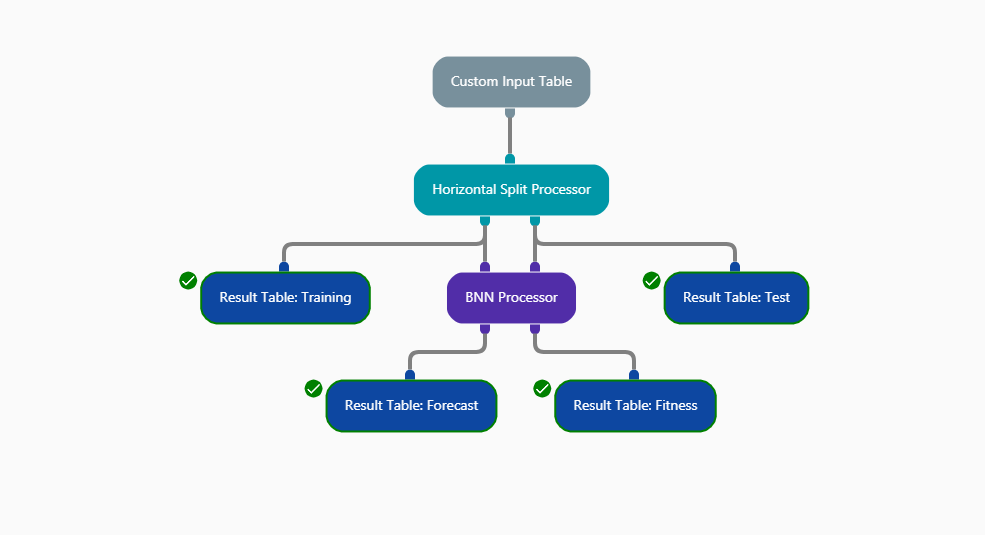Example workflow

Custom Input Table

The Custom Input Table Processor was used to generate the artificial dataset.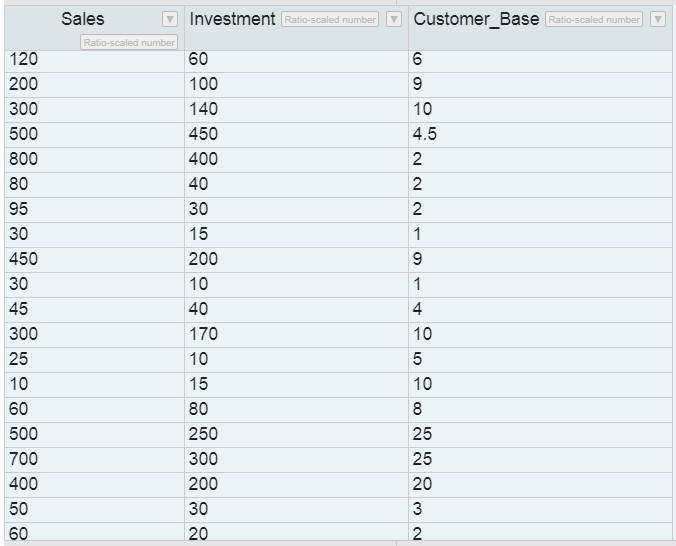Example artificial input dataset

Horizontal Split Processor

The Horizontal Split Processor was used to split the input dataset in test dataset and training dataset. The result of the Horizontal Split Processor can be inspected using the Result Table Processors.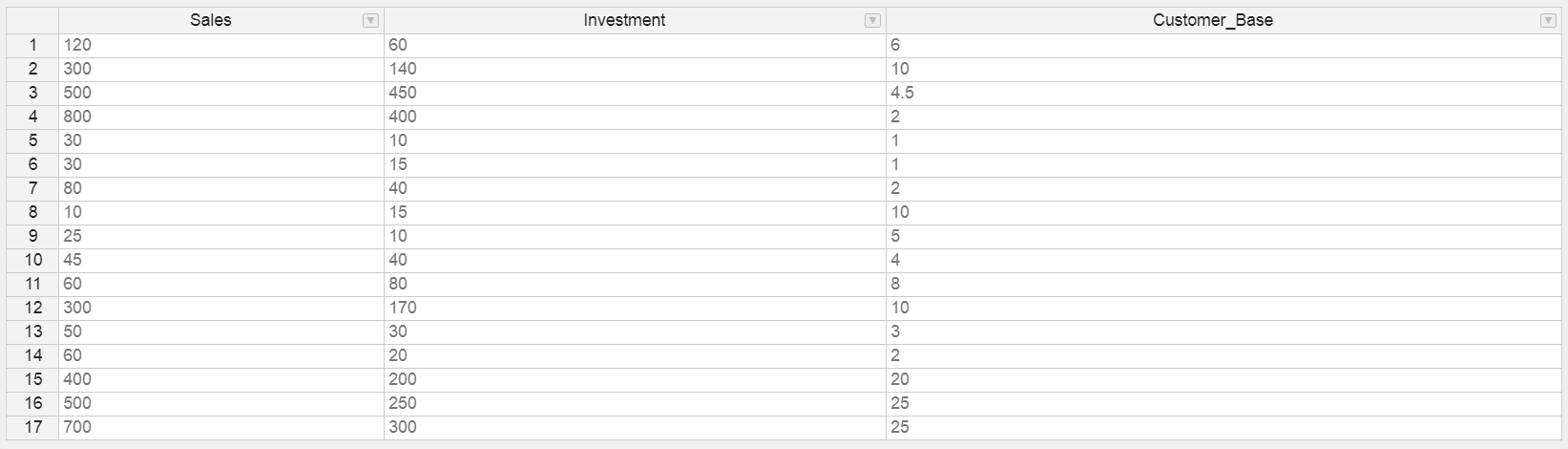Example input training dataset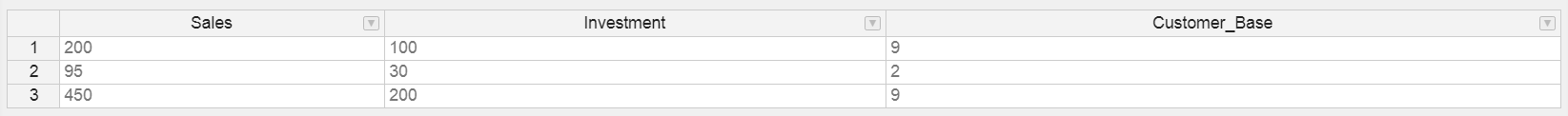Example input test dataset

BNN Processor

The configuration of the BNN processor for this example is given below. Most default configurations were left unchanged. We selected the "Sales" column as dependent column that should be predicted and the "Customer_Base" and "Investment" columns as independent columns used for prediction.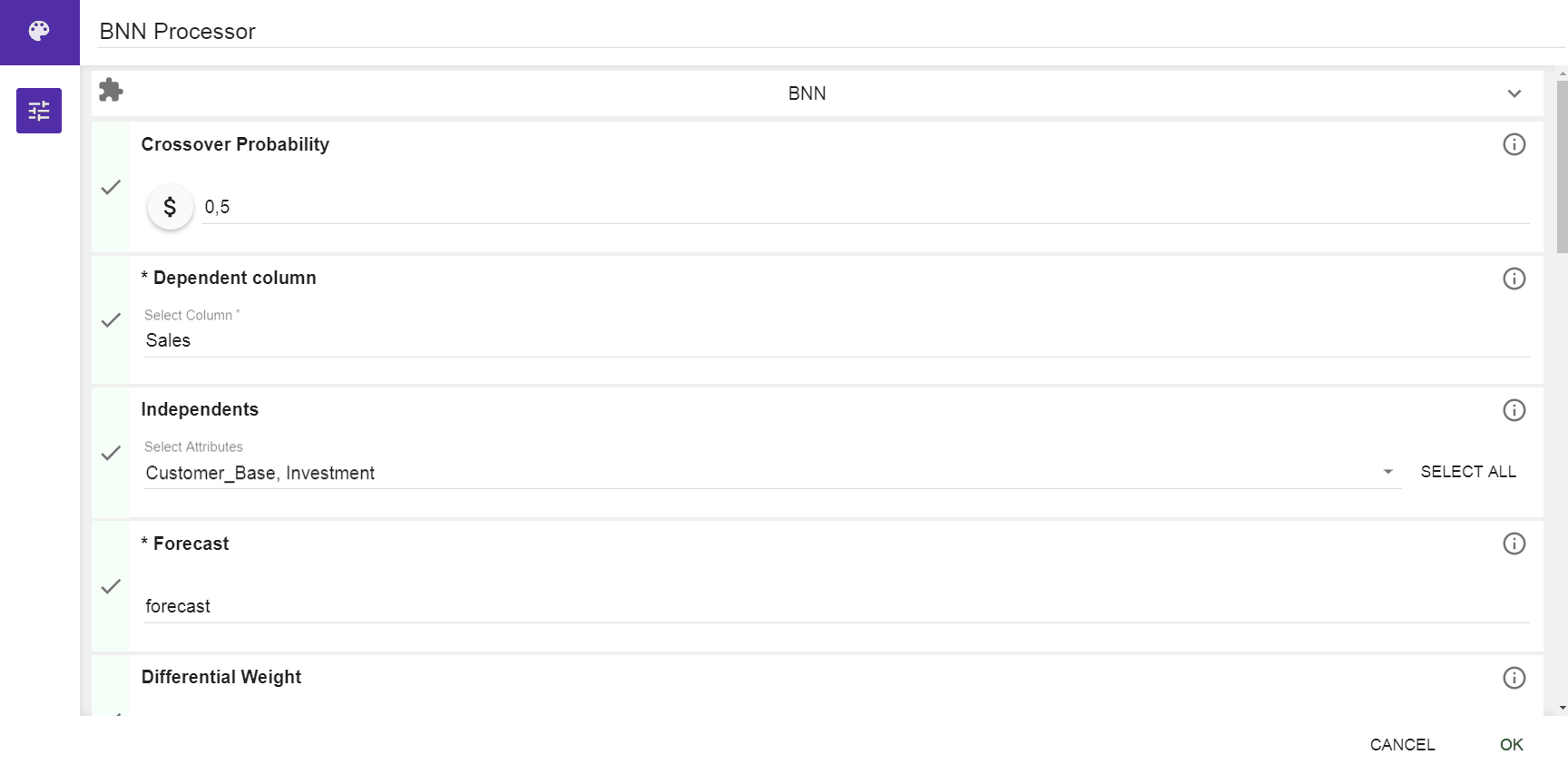Example configuration

Result

The BNN processor returns two result which can be inspected using the Result Table Processors.

The left output node returns the forecast for the test dataset.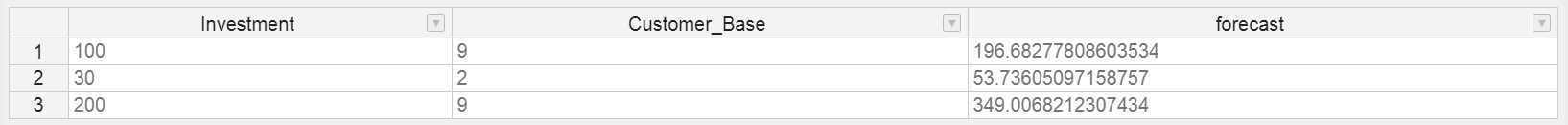Example output (left)

The right output node returns the fitness that was calculated for the model in each iteration. It is useful to inspect how the fitness improved in each iteration. At best the fitness should not change much in the last iterations of the algorithm. If there still are huge changes this might be an indication that the number of iterations should be increased.

The result of the altering fitness of the example above can be seen in the attachment "exampleoutput2.gif".